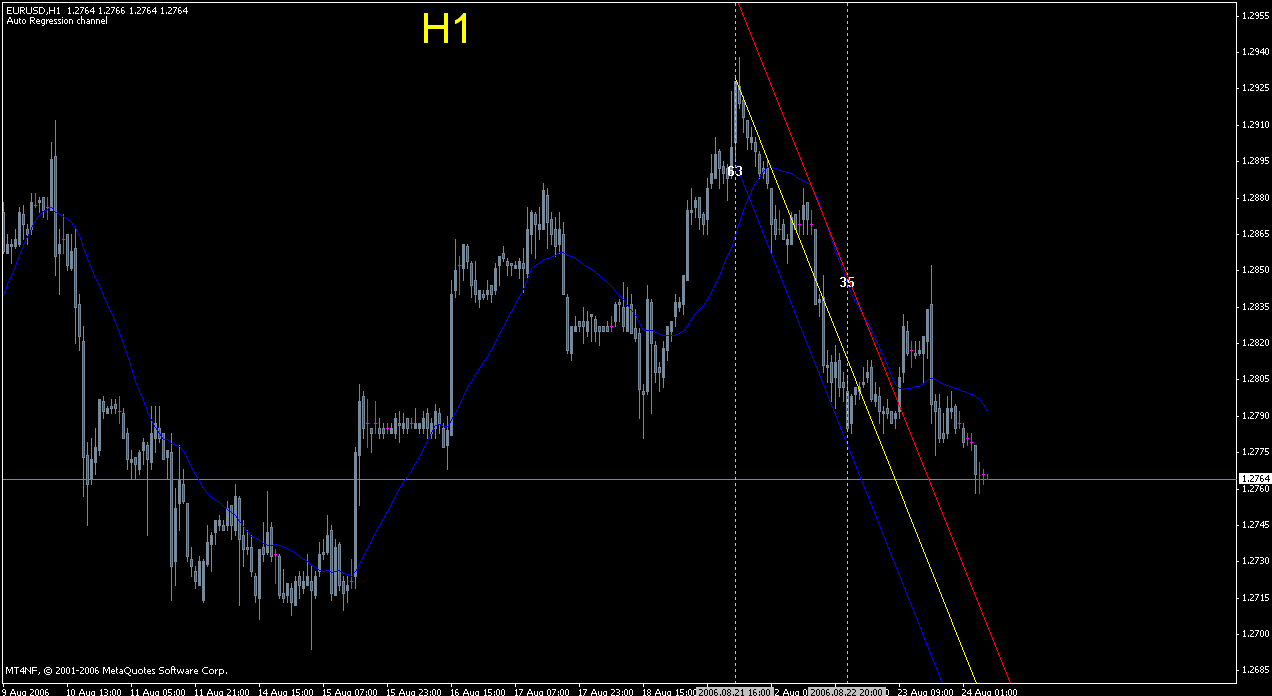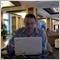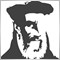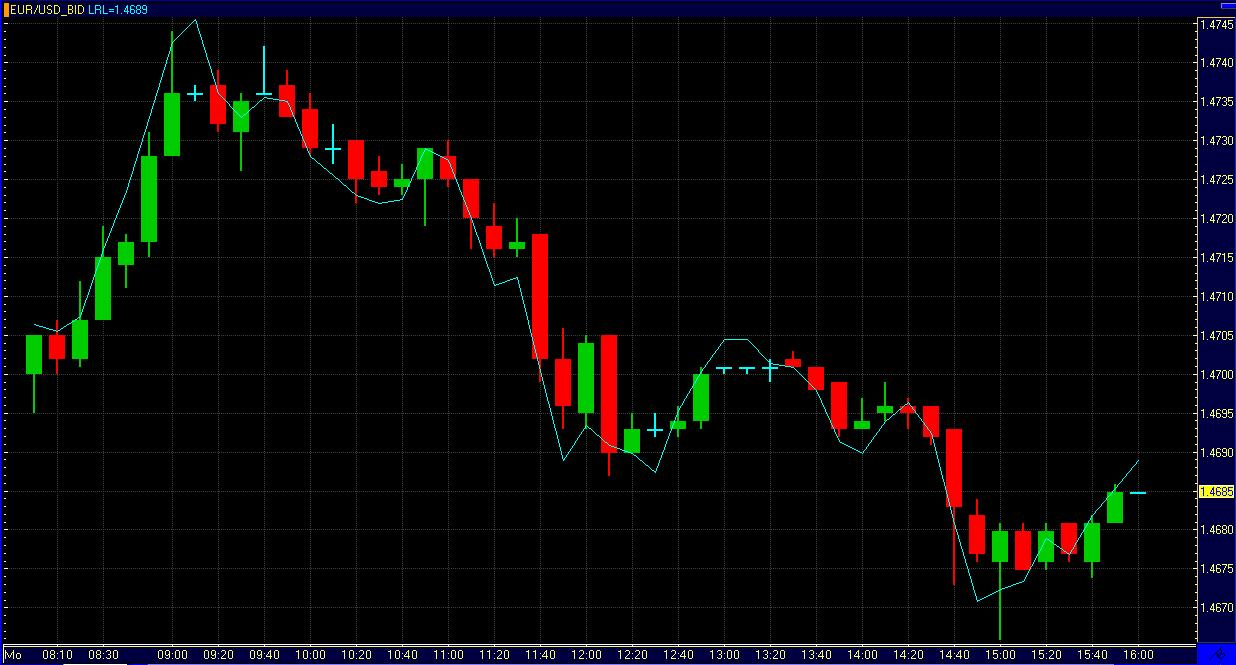Linear Regression Indicator - page 221

what is the number 63 & 35 in the image no.:2 , post no:2 stand for? Anyone know ?Another thing, how to make my image as thumbnail ?

http://img.photobucket.com/albums/v655/nak71/Marketiva/regressionh1.gifModerator
112949

As i understand the horizontal lines are 'Regression_begin" and "Regression_end". It means the indicator is drawing the channel from one line to the one one (horizontal line). But I don't know about numbers. Seems it is the price is crossing MA with period = 21 plus or minus something and this number is the numbers of bars starting from this crossing.42

Regression Indicators needed

Hi

i need Regression indicators for MT4 platform.below i placed all needed formulas and iformation of how to calcualte the indicators.plz make these for me and of course all members can use them also.any way here are the formulas :

****Linear Time Regression: Coefficient of Regression (Slope)****

Indicator Name : LinTimeRegSlope

Input Parameters:

TimeSeries=[Close or Open or High or Low]

RegressionPeriods= Integer Value(must be >= 3 )

Calculation:

[ n * sum(X*Y) - sum(X) * sum(Y) ] / [ n * sum(X*X) - sum(X) * sum(X) ]

where

sum(X*X) = sum of X*X over the last n periods

sum(X*Y) = sum of X*Y over the last n periods

sum(X) = sum of X over the last n periods

sum(Y) = sum of Y over the last n periods

X = Period Number

Y = Time Series

n = Regression Periods

****Linear Time Regression: Coefficient of Regression (Slope)****42

hi

**** Linear Time Regression: Coefficient of Correlation (r) ****

Indicator Name : "LinTimeRegr"

Input Parameters:

TimeSeries=[Close or Open or High or Low]

RegressionPeriods= Integer Value(must be >= 3 )

Calculation:

[ n * sum(X*Y) - sum(X) * sum(Y) ] /sqrt ( [n * sum(X*X) - sum(X) * sum(X)] * [n * sum(Y*Y) - sum(Y) * sum(Y)] )

where

sum(X*X) = sum of X*X over the last n periods

sum(Y*Y) = sum of Y*Y over the last n periods

sum(X*Y) = sum of X*Y over the last n periods

sum(X) = sum of X over the last n periods

sum(Y) = sum of Y over the last n periods

X = Period Number

Y = Time Series

n = Regression Periods

**** Linear Time Regression: Coefficient of Correlation (r) ****42

hi

here is 3rd one that uses 2nd indicator for calculaion based on below formula:

**** Linear Time Regression: Coefficient of Determination (r squared)****

Indicator Name : "LinTimeRegrsqrd"

Input Parameters:

TimeSeries=[Close or Open or High or Low]

RegressionPeriods= Integer Value(must be >= 3 )

Calculation:

LinTimeRegr(X, n) * LinTimeRegr(X, n)

where

X = Time Series

n = Regression Periods

**** Linear Time Regression: Coefficient of Determination (r squared)****5921

mimi:
Hi

i need Regression indicators for MT4 platform.below i placed all needed formulas and iformation of how to calcualte the indicators.plz make these for me and of course all members can use them also.any way here are the formulas :

Posts moved to this thread.

The forum have several thread Linear regression, some are:

https://www.mql5.com/en/forum/174257

https://www.mql5.com/en/forum/173057

https://www.mql5.com/en/forum/173215

https://www.mql5.com/en/forum/174257

and so on..167

Linear Regression

There is some function that returns the value of the earring of the straight "linear regression" of a certain period?

Might you help me?

Thank you.9

Linear Regression Indicator with Alert?

Does anyone know of a Linear Regression Indicator displaying channels with an alert where the latest bar hits either of the outside channel lines it sounds an alert?

If not, can someone with time on their hands revise the standard Linear Regression.mq4 indicator with such an alert.

Thanks in advance for all replies.

Scott6

MTF Linear Regression Channel

I am looking for a indi lrc for the longer time frames, Any ideas?6

Accucharts Linear Regression for Kelpie

i have been looking all over this forum and google and cant find a good Linear Regression indicator for mt4 that looks like the one on accuecharts.if someone can point me in the right direction or post the mq4 i would appreciate it.